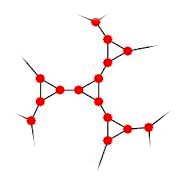# The Erdős–Gyárfás ConjectureCuriosity is a guiding light down the dark corridors of mathematical wonderment. With each turn, enlightenment protrudes from the ruble of faulty understanding into the illumination of new knowledge. With this analogy in mind, mathematics can aptly be described as a field in which a pencil, paper, and rational thought are employed to explore the connections and patterns embedded in the framework of algebraic and geometric abstractions. Theorem after theorem reveals the vast collection of mathematical discovery as well as the limitations of our current knowledge.

Therefore, it becomes apparent that epistemology provides a foundation for the mathematically curious to trudge. For instance, in the field of combinatorics, theory depends on what is known about countable discrete structures. One such structure can be found in the concept of a simple graph. In essence, a simple graph$G$ is a set of vertices and a set of edges (each with two ends) in which every edge has two vertices, exactly one at each end. As an example, a square is a simple graph in which the vertices are represented by the right angles where the edges meet.

If there is a connected simple graph with$n$ vertices, a cycle of length$n$ can be defined by starting at the$n$th vertex and traveling to the other$n-1$ vertices once and only once and returning to$n$th vertex. Suppose further, that there is one edge$e$ and a vertex$v$. If$v$ is an end vertex of$e$, then it is said that$e$ is incident to$v$. Moreover, the degree of a vertex$v$, denoted$deg(v)$, is the number of edges incident to$v$. The minimum degree of a graph is the number of edges incident to the vertex with minimum degree. In the example of the square, each vertex has degree 2. So, the minimum degree of the square (which is a cycle of length four) is 2.

One such open problem that deals with cycles and degrees in graphs is the Erdős–Gyárfás Conjecture, proposed in 1995 by mathematicians Paul Erdős and András Gyárfás. The formal statement is as follows:

The Erdős–Gyárfás Conjecture: Every graph with minimum degree 3 contains a simple cycle whose length is a power of 2.

A stronger version would be to prove the conjecture true for every cubic graph.  That is, for every graph in which every vertex is of degree 3. If a counterexample is to be constructed, the resultant graph would have at least 17 vertices as verified by computation.   A quick internet search will yield a brief summary of the current research and computational results.   How would you construct a counterexample or proof?  It only takes a little curiosity to light the way.